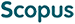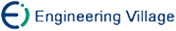张发存, 赵晓红, 王 忠, 沈绪榜. 面向算法的SIMD计算机数学模型及其应用研究[J]. 计算机研究与发展, 2005, 42(4): 557-562.
 引用本文: 张发存, 赵晓红, 王 忠, 沈绪榜. 面向算法的SIMD计算机数学模型及其应用研究[J]. 计算机研究与发展, 2005, 42(4): 557-562.Zhang Facun, Zhao Xiaohong, Wang Zhong, Shen Xubang. Study of Algorithm-Oriented Mathematical Model of SIMD Computer and Its Applicat ion[J]. Journal of Computer Research and Development, 2005, 42(4): 557-562.
 Citation: Zhang Facun, Zhao Xiaohong, Wang Zhong, Shen Xubang. Study of Algorithm-Oriented Mathematical Model of SIMD Computer and Its Applicat ion[J]. Journal of Computer Research and Development, 2005, 42(4): 557-562.## Study of Algorithm-Oriented Mathematical Model of SIMD Computer and Its Applicat ion

• 摘要: 针对数据并行计算在图像处理中的应用研究，提出了数据并行计算机的面向算法的数学模型，以及利用该模型得到的一种新颖的、数据并行算法的数学描述方法.采用该数学描述方法 对数据并行图像处理中的灰度直方图运算、区域增长法图像分割以及图像卷积运算等3类图 像处理方法进行了描述.结果表明，该数学描述方法不仅简单可行和精确，而且，可以从数 学公式中直接得到算法的通信复杂性和计算复杂性.该方法可以应用到数据并行计算的应用 研究中作为数学描述的工具.

Abstract: By the study of image processing in the field of data parallel computation, an algorithm-oriented mathematical model of SIMD computer, and a novel method which is useful for mathematical description of data parallel algorithm are proposed. By using the method proposed, detailed descriptions of data parallel algorithm o n histogram operation, region-growing image segmentation and image convolution o peration are given. This mathematical description method is simple and accurate, the communication complexity and computation complexity of the algorithm being studied can be obtained easily in the mathematical formula. The method can be us ed as mathematical description in applications of data parallel algorithm studie s./下载:  全尺寸图片 幻灯片
• 分享
• 用微信扫码二维码

分享至好友和朋友圈